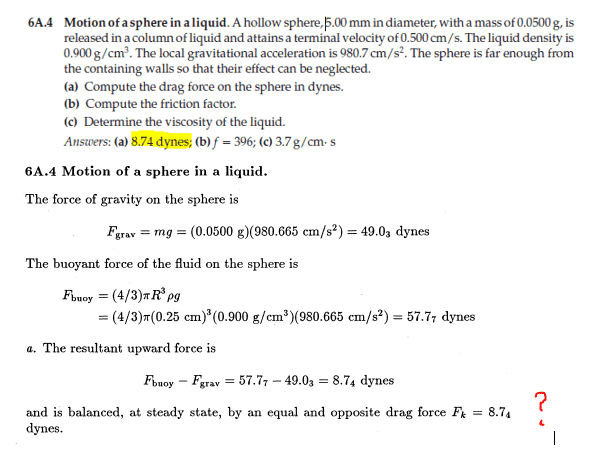# Motion of a sphere in a liquid

Est120
Homework Statement:
determine the drag force
Relevant Equations:
Archimedes principle formula, weight formula
in my head this is just a silly problem in which i need to determine the ↓ force (weight) and the ↑force (archimedes bouyant force) and then the difference must be the drag force ↑ (the force that involves velocity) but i can't get any sense out of this answer
how is possible for the sphere to reach terminal velocity (and thus keep falling) if the bouyant force is greater in magnitude than the weight?
how is the drag force acting downwards (in the direction of motion) ? this doesn't make any sense to me